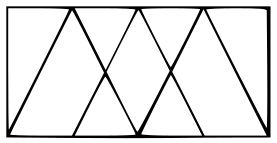Question 2

# How many triangles are there in the given figure ?Solution

Small triangles = ABF, BKC, GKH, CLD, HLI, DEJ

Big triangles = BFH, DHJ, GCI, BDH

Thus, total triangles = 10

=> Ans - (B)